UPSC  >  Basic Concept: Profit, Loss & Discount

# Basic Concept: Profit, Loss & Discount Notes | Study CSAT Preparation for UPSC CSE - UPSC

## Document Description: Basic Concept: Profit, Loss & Discount for UPSC 2022 is part of CSAT Preparation for UPSC CSE preparation. The notes and questions for Basic Concept: Profit, Loss & Discount have been prepared according to the UPSC exam syllabus. Information about Basic Concept: Profit, Loss & Discount covers topics like Introduction, Profit, Loss, and Discount, Profit and Loss Common Formulas, Profit and Loss Tricks, Some Solved Problems and Basic Concept: Profit, Loss & Discount Example, for UPSC 2022 Exam. Find important definitions, questions, notes, meanings, examples, exercises and tests below for Basic Concept: Profit, Loss & Discount.

Introduction of Basic Concept: Profit, Loss & Discount in English is available as part of our CSAT Preparation for UPSC CSE for UPSC & Basic Concept: Profit, Loss & Discount in Hindi for CSAT Preparation for UPSC CSE course. Download more important topics related with notes, lectures and mock test series for UPSC Exam by signing up for free. UPSC: Basic Concept: Profit, Loss & Discount Notes | Study CSAT Preparation for UPSC CSE - UPSC
 Table of contents Introduction Profit, Loss, and Discount Profit and Loss Common Formulas Profit and Loss Tricks Some Solved Problems
 1 Crore+ students have signed up on EduRev. Have you?

Introduction

• The profit and Loss formula is used in mathematics to determine the price of a commodity in the market and understand how profitable a business is.
• Every product has a cost price and a selling price.
• Based on the values of these prices, we can calculate the profit gained or the loss incurred for a particular product.

Profit, Loss, and Discount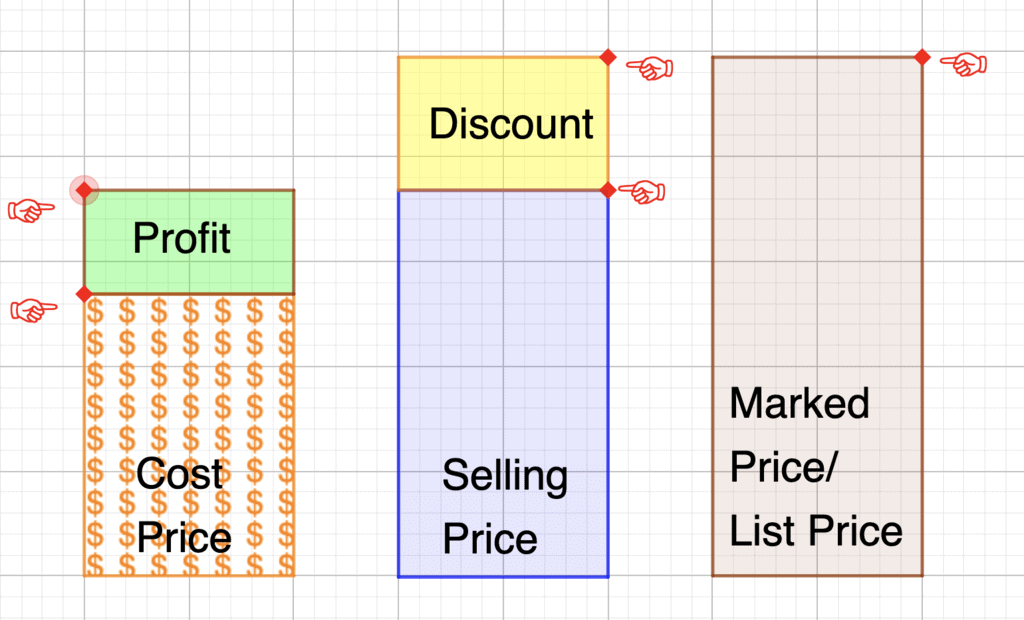1. Cost Price (CP)

The amount paid for a product or commodity to purchase it is called a cost price. Also, denoted as CP.
This cost price is further classified into two different categories:

• Fixed Cost: The fixed cost is constant, it doesn’t vary under any circumstances
• Variable Cost: It could vary depending as per the number of units

2. Selling price (SP)

• The amount for which the product is sold is called Selling Price. It is usually denoted as SP. Also, sometimes called a sale price.

3. Profit and Loss

Profit: When an article is sold for more than the cost of the article, then we say there is a Profit or Gain.

• Profit or Gain = S.P - C.P

Loss: When an article is sold for less than the cost of the article,  then we say there is a Loss.

• Loss = C.P - S.P

The formula for the profit and loss percentage is:

• Profit percentage = (Profit / Cost Price) x 100
• Loss percentage = (Loss / Cost price) x 100

4. Marked Price Formula (MP)

This is basically labeled by shopkeepers to offer a discount to the customers in such a way that,

• Discount = Marked Price – Selling Price
• Discount Percentage = (Discount/Marked price) x 100

Profit and Loss Common Formulas

You have learned until now how to calculate profit as well as loss and also the percentage of them. Here is a list of the most commonly used formulas for all related questions: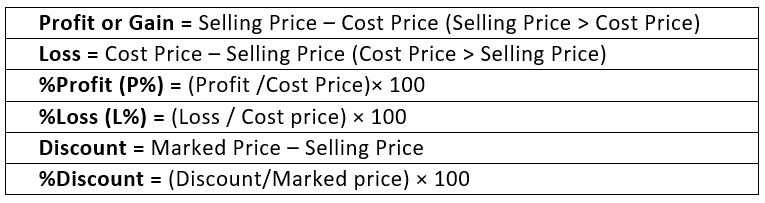Profit and Loss Tricks

Now let us look at some additional tricks or formulas that can be used to solve maths problems based on gain and loss:

• SP = {(100 + P%)/100} x CP
• SP = {(100 – L%)/100} x CP
• CP = {100/(100 + P%)} x SP
• CP = {100/(100 – L%)} x SP
• SP = MP - Discount
• For false weight, profit percentage will be P% = (True weight – false weight/ false weight) x 100.
• When there are two successful profits say m% and n%, then the net percentage profit equals to (m+n+mn)/100
• When the profit is m% and loss is n%, then the net % profit or loss will be: (m-n-mn)/100
• If a product is sold at m% profit and then again sold at n% profit then the actual cost price of the product will be: CP = [100 x 100 x P/(100+m)(100+n)].
In case of loss, CP = [100 x 100 x P/(100-m)(100-n)]
• If P% and L% are equal then, P = L and %loss = P2/100

Some Solved Problems

To understand the concept let's first open a shop say a local retail stationery shop.

Example 1: Now suppose a student shows up and wants to buy 5 gel pens worth ₹ 5 each. So, what is the profit earned by the shopkeeper in net terms and its profit percentage?

This ₹ 5 is the selling price, the price at which the commodity is sold to its buyer. And let ₹ 4 is the price at which the shopkeeper has bought these pens from his supplier and this price is known as Cost Price.
Thus, this difference between the price at which the shopkeeper buys his pen and at which it is sold is known as profit/ loss earned. Now if Selling Price > Cost Price then he will earn profit and if Selling Price < Cost Price, then he will earn loss.
Therefore, Profit = Selling Price – Cost Price ₹ 5 – ₹4 = ₹1 per pen
Now,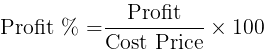i.e.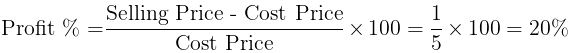And consequently, Loss = Cost Price – Selling Price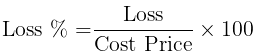i.e.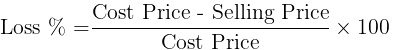Example 2: Suppose another customer comes to the shop and bought 2 registers worth ₹ 50 each and a pencil box from him. And this time the shopkeeper has earned 40% profit on the registers. He earned a profit of ₹10 on pencil box and the profit% on pencil box is 20%. Then what is the cost price and profit on register and selling price and cost price of pencil box?

In this case, we are given S.P. = ₹ 50 for each register and profit% =  40%. Let C.P. be x.
⇒ Profit % = {(50 – x)/x} * 100
⇒ 40 = {(50 – x)/x} * 100
⇒ 4x = 500 – 10x
⇒ 14x = 500
⇒ x = 35.71
⇒ Profit = 50 – 35.71 = 14.83

What if the profit of 40% is on selling price instead?
⇒ Profit = profit% * S.P = 0.4* 50 = ₹20
C.P. = S.P. – Profit = ₹50 – ₹20 = ₹30

Let's now move on to calculate S.P. and C.P. of pencil box. Let C.P. of pencil box be y
∵ Profit % = (profit/ Cost price) *100
⇒ 20 = (10/y) *100
⇒ 2y = 100
⇒ y = ₹ 50
Therefore, S.P. = ₹50 + ₹10 = ₹60

Question for Basic Concept: Profit, Loss & Discount
Try yourself:Alfred buys an old scooter for Rs. 4700 and spends Rs. 800 on its repairs. If he sells the scooter for Rs. 5800, his gain percent is:

Example 3: Since not many customers showed up on the first day of the shop. Therefore, to popularize the shop the shopkeeper puts up discount of 20% on all the products. The first customer shows up and bought a packet of pencils and 3 erasers and still making up the profit of 30% on both the items. Then what is the actual cost price of both the items when the pencil is marked as ₹30 and the eraser ₹ 5 each?

What does the underlined marked mean?

Here marked means Marked Price is the price that is offered to customer before discount basically, discount is just difference between marked price and Selling price i.e. Discount = M.P. – S.P.

In this case, Discount = 0.2 * Price of packet of pencil = 0.2 *30 = ₹ 6
Therefore, S.P = 30 – 6 = ₹ 24
∵ Profit is 30% on C.P., assume C.P. be x
⇒ 0.3 = (24 – x)/x
⇒ 0.3x = 24 – x
⇒ 1.3x = 24
⇒ x = 18.46
Similarly,
Discount = 0.2 * Price of 3 erasers = 0.2* 3*5 = ₹ 3
Hence, S.P. = (3*5) - 3 = ₹ 12
∵ Profit is 30% on C.P., assume C.P. be y
⇒ 0.3 = (12 – y)/y
⇒ 1.3y = 12
⇒ y (C.P. of 3 eraser) = 9.23
The above method of solving questions was a direct straightforward way of finding the solution. There's also an alternative way of doing so. Let's now learn that alternative method but this time instead of earning profit the seller was earning the loss.

Example 4: Consider this situation, that the stationery sold a parker pen at a loss of 20% for ₹ 100 and a pack of colored sketch pens at the loss of 15% on S.P.  What are the cost price and selling price of both the articles?

First, let's find out the C.P. and S.P. parker pen,
∵ Loss is 20% on C.P.
Let C.P. be x and S.P. be 100
⇒ S.P. = 0.8 of C.P.
⇒ 100 = 0.8x
⇒ 100/0.8 = x
⇒ 125 = x
For sketch pens,
∵ Loss is 15% on S.P.
Let S.P. be y and C.P. be 100
⇒ Loss = 0.15y
⇒ C.P. = 1.15 y
⇒ 100 = 1.15y
⇒ 100/1.15 = y
⇒ y = ₹ 87

Question for Basic Concept: Profit, Loss & Discount
Try yourself:The cost price of 20 articles is the same as the selling price of x articles. If the profit is 25%, then the value of x is:

The problems explained above are rather simple and easy ones. Now let's do some problems that are bit complex and have come up in the previous year's CAT exam.

Example 5: A watch dealer incurs an expense of Rs. 150 for producing every watch. He also incurs an additional expenditure of Rs. 30,000, which is independent of the number of watches produced. If he is able to sell a watch during the season, he sells it for Rs. 250. If he fails to do so, he has to sell each watch for Rs. 100. If he is able to sell only 1,200 out of 1,500 watches he has made in the season, then he has made a profit of:
(a) ₹ 90000
(b) ₹ 75000
(c) ₹ 45000
(d) ₹ 60000

This question is not basic and direct as the problems given above. This one came in CAT 2016 paper and you should expect to get this level of questions in the exam and not the ones explained above earlier. Now let's see how to solve this problem

Here, first find out cost he has incurred to produce the watches.
∵ He made 1500 watches costing ₹ 150 each and an additional ₹ 30000 expense on them.
⇒ Total Cost = (1500*150) + 30000 = ₹ 255000
∵ He's able to sell 1200 watches in the season = ₹250 each
So, the revenue earned by him during the season = ₹250 * 1200 = ₹ 300000
Also, the leftover 300 pieces of clocks would have been sold by the watchmaker in off-season = 100 each.
Revenue earned through these 300 watches = 300*100 = ₹ 30000
Total Revenue = ₹ 300000 + ₹30000 = ₹330000
Profit = Revenue - Cost = 330000 - 255000 = ₹ 75000

Question for Basic Concept: Profit, Loss & Discount
Try yourself:A vendor bought toffees at 6 for a rupee. How many for a rupee must he sell to gain 20%?

Example 6: Instead of a meter scale, a cloth merchant uses a 120cm scale while buying, but uses an 80cm scale while selling the same cloth. If he offers a discount of 20% on cash payment, what is his overall profit percentage?

This question above is a special one with the faulty dealer. Here, the dealer is earning profit by using a false scale.
To solve this problem, first assume that the price of the cloth is ₹ 1/cm
∵ He's using a 120 cm scale.
⇒ C.P. = (100/120) * ₹1 = ₹ 0.8333/cm
∵ This merchant again uses faulty scale to sell the cloth to his customers. He uses a scale that measures 80cm as 100cm i.e. he sells 80cm for ₹100
Now he also gives a discount of 20% on the cloth.
⇒ His mark up price is ₹100/80cm
∵ S.P. = M.P. - M.P. * Discount% = M.P. (1 - Discount%) = (100/80)*(80/100) = ₹1/cm
Therefore, his profit % = (1 – 0.8333)/0.8333 *100 = 20%

Question for Basic Concept: Profit, Loss & Discount
Try yourself:When a plot is sold for Rs. 18,700, the owner loses 15%. At what price must that plot be sold in order to gain 15%?

The document Basic Concept: Profit, Loss & Discount Notes | Study CSAT Preparation for UPSC CSE - UPSC is a part of the UPSC Course CSAT Preparation for UPSC CSE.
All you need of UPSC at this link: UPSC

## CSAT Preparation for UPSC CSE

72 videos|64 docs|92 tests
 Use Code STAYHOME200 and get INR 200 additional OFF

## CSAT Preparation for UPSC CSE

72 videos|64 docs|92 tests

### How to Prepare for UPSC

Read our guide to prepare for UPSC which is created by Toppers & the best Teachers

Track your progress, build streaks, highlight & save important lessons and more!

,

,

,

,

,

,

,

,

,

,

,

,

,

,

,

,

,

,

,

,

,

,

,

,

;